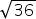Mathematics
Easy

Question

# Rewriteinto simplest form

## 5678Hint:

## The correct answer is: 6

### Here, we have to write the simplest form of √36 .Now, √36 = √ ( 2 × 2 × 3 × 3 )= √ 22 × 32 = 2 × 3= 6Hence, the correct option is (b).

The square root is an inverse method of squaring a number.

### Related Questions to study#### With Turito Foundation.#### Get an Expert Advice From Turito.